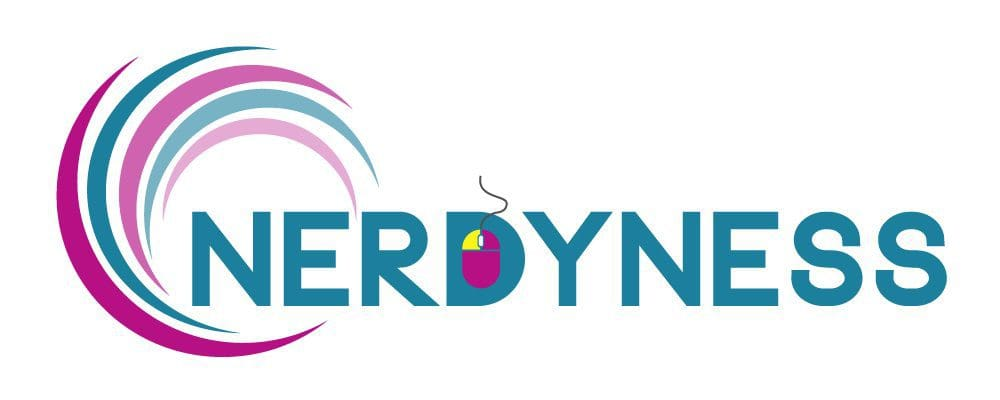# Nerdyness Designer KPIs Form

• From the below options, choose the one that fits the most
• From the below options, choose the one that fits the most
• From the below options, choose the one that fits the most
• From the below options, choose the one that fits the most
• From the below options, choose the one that fits the most
• From the below options, choose the one that fits the most
• From the below options, choose the one that fits the most
• From the below options, choose the one that fits the most
• From the below options, choose the one that fits the most
• From the below options, choose the one that fits the most
• From the below options, choose the one that fits the most
• From the below options, choose the one that fits the most
• From the below options, choose the one that fits the most
• From the below options, choose the one that fits the most